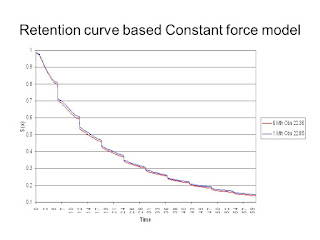Posts

Showing posts from April, 2018

CFM vs UDD vs BalducciLife Tables: It is a computational tool based on a specific survival model. Our task is to generate a survival model and our output will be a life table. Life table is based on Unitary method. lx= Expected no. of lives at age x dx= Expected no. of deaths between exact age x and exact age x+1 NOTE: We call it expected and not actual because most of the values will be in decimal too, so how can it be actual.
But there is a limitation of Life Tables: It is defined only for integer ages. If we have to calculate probability of death/survival at any non-integer age we will use 3 assumptions: 1.CFM= Constant Force of Mortality 2.UDD= Uniform Distribution of death 3.Balducci assumption
1.CFM ·It assumes that the force of mortality is constant, i.e. ux+t = ux ·If the force of mortality of a newborn is constant, it means that the expected future lifetime of this life is 1/μ no matter what age he is in. ·Survival Function in this case will decrease exponentially. ·If 0<s<t<1 then t-spx+s = tpx/spx …